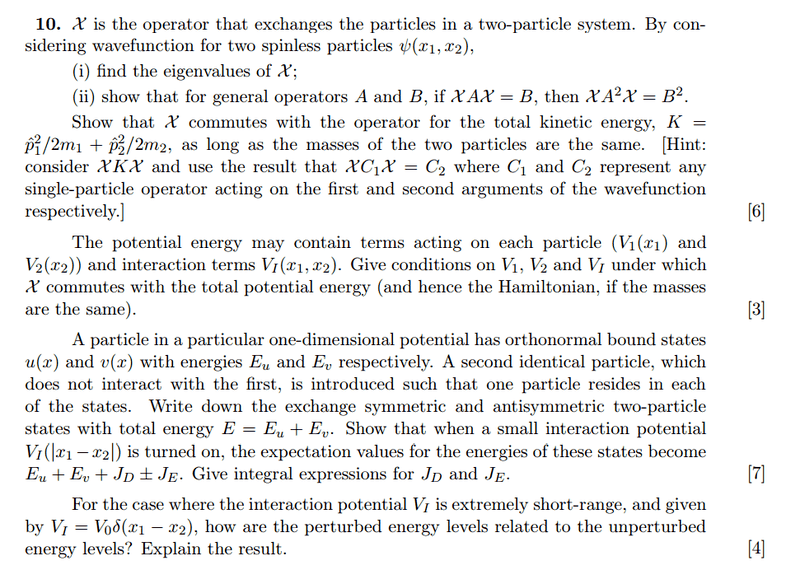# Perturbation Theory, exchange operator

## Homework StatementPart (a): Find eigenvalues of X, show general relation of X and show X commutes with KE.
Part (b): Give conditions on V1, V2 and VI for X to commute with them.
Part (c): Write symmetric and antisymmetric wavefunctions. Find energies JD and JE.
Part (d): How are unperturbed and perturbed energy levels related?

## The Attempt at a Solution

Part (a)
The eigenvalues I found are ##\pm 1##, which seems right.

No problem with this showing X commutes with K too.

Part(b)
I'm thinking X will commute with ##x^2##, so this means that V1 and V2 must be functions of ##x^2##.
As for VI, I'm not sure.

Part (c)

For two particles A and B,
Symmetric wavefunction is:

$$\frac{1}{\sqrt 2} \left(|u,A>|v,B> + |v,A>|u,B>\right)$$

Anti-Symmetric wavefunction is:

$$\frac{1}{2} \left( |u,A>|v,B> - |v,A>|u,B>\right)$$

I'm not sure what ##J_D## and ##J_E## are. I'm guessing ##J_E## is the first order perturbed energy ##<E|V_I|E>##?

Part (d)

The delta function means that ##x_1 = x_2##, so the perturbed energy levels is the same as unperturbed? Not sure why either.

I'm guessing ##J_D## is the interaction energy, ##J_E## is the first order perturbed energy. So this perturbation "lifts" the degeneracy.

Isn't ##J_D = V_{|x_1-x_2|}## while ##J_E = <s+|V_I|s+>## where ##|s+>## is the symmetric wavefunction?

How do I overlap this symmetric wavefunction: ##\frac{1}{\sqrt 2} \left(|u,A>|v,B> + |v,A>|u,B>\right)##?
Since ##u_{(x)}## and ##v_{(x)}## are orthonormal states, can I assume ##<u,A|v,A> = <u,B|v,B> = 0##?

Therefore ##\langle\psi_s|\psi_s\rangle = \frac{1}{2}\int \langle u,A|u,A\rangle\langle v,B|v,B\rangle + \langle v,A|v,A\rangle\langle u,B|u,B\rangle d^3r = \int 2|u|^2|v|^2 d^3r##?

Last edited:
bumpp